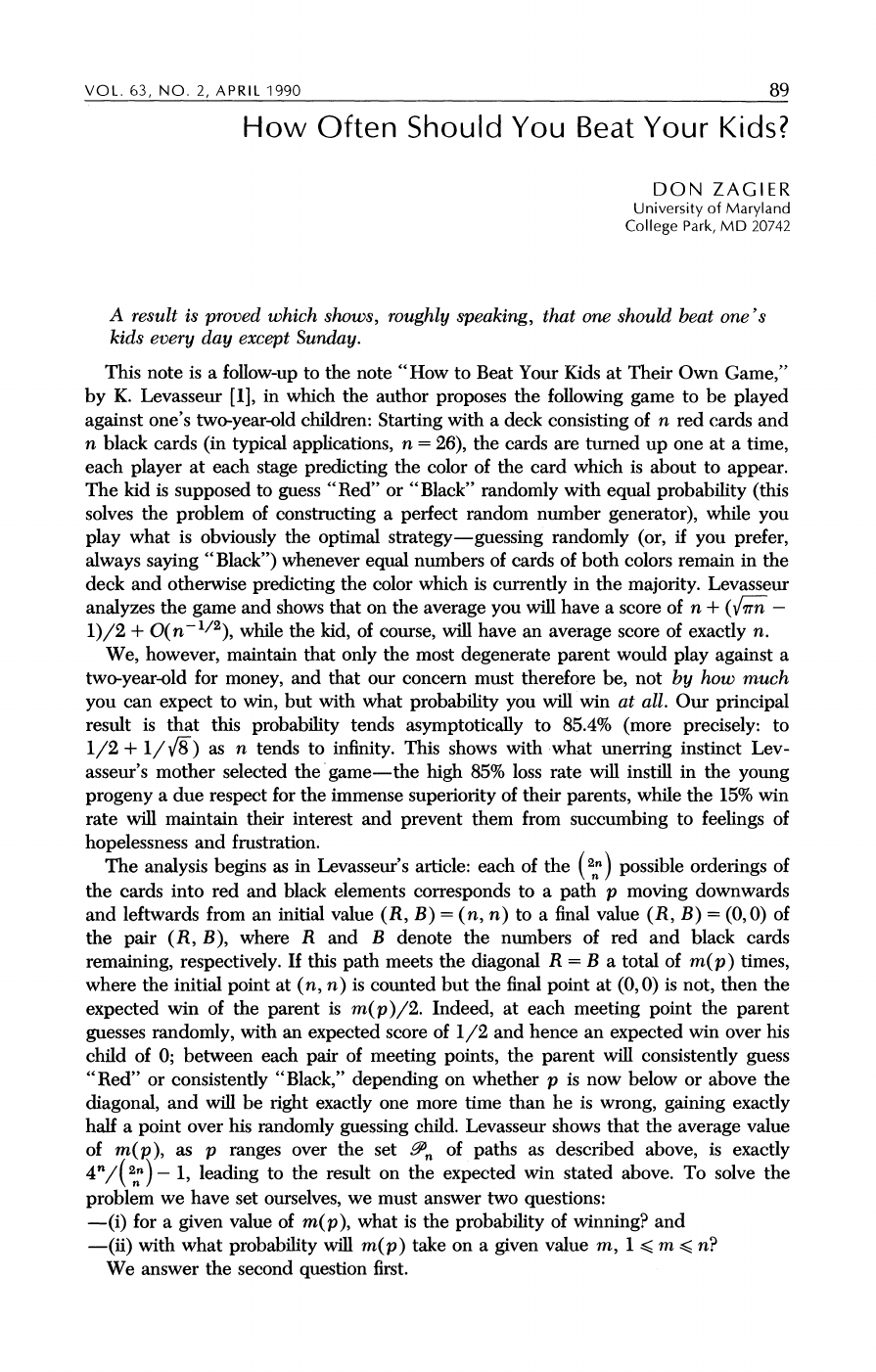Don Zagier, born in 1951, is an American mathematician whose main a...
Here's a link to [Levasseur's original paper](https://fermatslibrar...
A game path in Levasseur's paper is determined by the position of $... To understand the expected wins for both the kid (👶🏻) and the paren... A way to prove that$\sum_{n=0}^{\infty} \binom{2n}{n} x^n = \frac...
This integral is called the Gaussian integral. To solve it we not...
There's an interesting variation of this problem in which the numbe...VOL. 63, NO. 2, APRIL 1990
89
How
Often Should You Beat Your Kids?
DON ZAGIER
University
of
Maryland
College Park,
MD 20742
A
result is proved which
shows, roughly speaking, that one should beat one's
kids every day except Sunday.
This note is a follow-up to the
note "How to Beat Your Kids at Their Own Game,"
by
K.
Levasseur
,
in
which the
author
proposes
the
following game to be played
against
one's
two-year-old
children:
Starting
with a
deck consisting of
n
red cards and
n
black cards (in typical
applications,
n
=
26), the cards are turned up one at a time,
each
player
at each
stage
predicting
the color of the card which is
The kid is supposed to guess "Red"
or
"Black"
randomly
with
equal probability (this
solves the
problem
of
constructing
a
perfect
random number
generator),
while
you
play what is obviously the optimal
strategy-guessing randomly (or,
if
you prefer,
always saying "Black")
whenever
equal
numbers
of
cards of both colors remain
in
the
deck
and
otherwise
predicting
the color
which
is
currently
in
the
majority.
Levasseur
analyzes the game
and shows that
on
the
average you
will
have a score of
n
+
(VFW
-
1)/2
+
O(n- 1/2),
while the
kid,
of
course,
will
have
an average score
of
exactly
n.
We, however, maintain that
only
the
most
degenerate parent
would
play against
a
two-year-old
for
money,
and
that
our concern
must therefore
be,
not
by
how much
you
can
expect
to
win,
but
with
what
probability you
will win
at all.
Our
principal
result is that this
probability tends
asymptotically to 85.4% (more precisely: to
1/2
+
1/
F8)
as
n
tends to
infinity.
This
shows
with
what unerring instinct Lev-
asseur's mother selected the
game-the
high
85%
loss rate
will
instill
in
the
young
progeny
a
due respect
for the
immense superiority
of
their parents,
while
the
15%
win
rate will
maintain
their
interest
and
prevent them
from
succumbing
to
feelings
of
hopelessness
and
frustration.
The
analysis begins
as
in
Levasseur's article:
each of
the
(2n)
possible
orderings
of
the cards
into
red and black elements
corresponds
to
a
path p moving
downwards
and leftwards from
an initial value
(R, B)
=
(n, n)
to a
final value
(R, B)
=
(0, 0)
of
the
pair (R, B),
where
R
and
B
denote the numbers
of red and
black
cards
remaining, respectively.
If this
path
meets the
diagonal
R
=
B
a
total
of
m(p)
times,
where the initial
point
at
(n, n)
is counted but the final
point
at
(0, 0)
is
not,
then the
expected
win of
the
parent
is
m(p)/2. Indeed,
at each
meeting point
the
parent
guesses randomly,
with
an
expected
score
of
1/2
and
hence an
expected
win
over
his
child of
0;
between each
pair
of
meeting points,
the
parent
will
consistently guess
"Red" or
consistently "Black,"
depending
on whether
p
is
now
below or above
the
diagonal,
and
will
be
right exactly
one more time than he is
wrong, gaining exactly
half a
point
over
his
randomly
guessing
child. Levasseur shows
that the
average
value
of
m(p),
as
p ranges
over the set
;n
of
paths
as described
above,
is
exactly
to the result
on the
expected
win
stated above. To solve
the
problem
we have set
ourselves,
we
must
questions:
-(i)
for a
given
value of
m(p),
what
is
the
probability
of
winning?
and
-(ii)
with what
probability
will
m(p)
take
on a
given
value
m,
1
<
m
<
n?
We